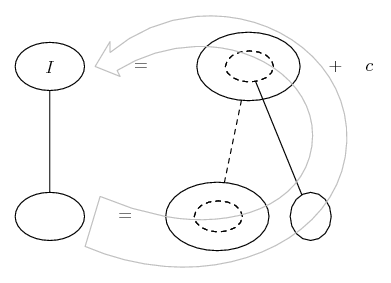# Thread: Intergral

1. ## Intergral

Hi, got a question about the intergral of e^x+1/e^x-1

I put u=e^x-1, dx=du/e^x
And i am left with the intergral of 1/u+1, which isnt right. Can anyone help me?

2. Yes, if you let $\displaystyle u= e^x- 1$ then $\displaystyle du= e^xdx$ so that $\displaystyle dx= du/e^x= du/(u+ 1)$.
Also $\displaystyle e^x+ 1= u+ 2$.

But then $\displaystyle \int \frac{e^x+ 1}{e^x- 1}dx= \int \frac{u+ 2}{u}(u+1)du= \int\frac{(u+2)(u+1)}{u}du$ which is certainly NOT "1/(u+1)".

3. But if dx=du/u+1, then the intergral should be u+2/u(u+1) and not (u+2)(u+1)/u, right?

4. Yes, I think that was a typo/slip. Just in case a picture helps - in confirming your reasoning re the typo but then also getting past your alleged 1/(u+1)...... where (key in spoiler) ...

Spoiler:... is the chain rule. Straight continuous lines differentiate downwards (integrate up) with respect to the main variable (in this case x), and the straight dashed line similarly but with respect to the dashed balloon expression (the inner function of the composite which is subject to the chain rule).

The general drift is..._________________________________________

Don't integrate - balloontegrate!

Balloon Calculus; standard integrals, derivatives and methods

Balloon Calculus Drawing with LaTeX and Asymptote!

#### Search Tags

intergral